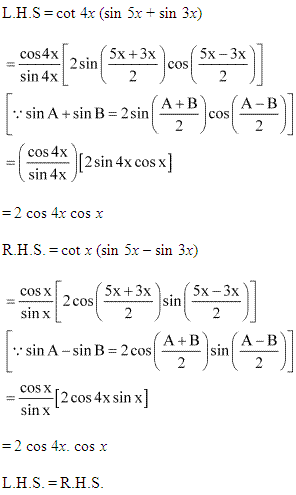# NCERT Solutions for Class 11-science Maths Chapter 3 - Trigonometric Functions

Page / Exercise

## Chapter 3 - Trigonometric Functions Ex. 3.1

Solution 1Solution 2Solution 3Solution 4Solution 5Solution 6Solution 7## Chapter 3 - Trigonometric Functions Ex. 3.2

Solution 1Solution 2Solution 3Solution 4Solution 5Solution 6Solution 7Solution 8Solution 9Solution 10## Chapter 3 - Trigonometric Functions Ex. 3.3

Solution 1Solution 2Solution 3Solution 4Solution 5Solution 6Solution 7Solution 8Solution 9Solution 10Solution 11Solution 12Solution 13Solution 14Solution 15Solution 16Solution 17Solution 18Solution 19Solution 20Solution 21Solution 22Solution 23Solution 24Solution 25## Chapter 3 - Trigonometric Functions Ex. 3.4

Solution 1Solution 2Solution 3Solution 4Solution 5Solution 6Solution 7Solution 8Solution 9## Chapter 3 - Trigonometric Functions Misc. Ex.

Solution 1Solution 2Solution 3Solution 4Solution 5Solution 6Solution 7Solution 8Solution 9Solution 10### STUDY RESOURCES

REGISTERED OFFICE : First Floor, Empire Complex, 414 Senapati Bapat Marg, Lower Parel, Mumbai - 400013, Maharashtra India.